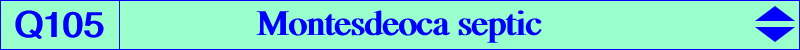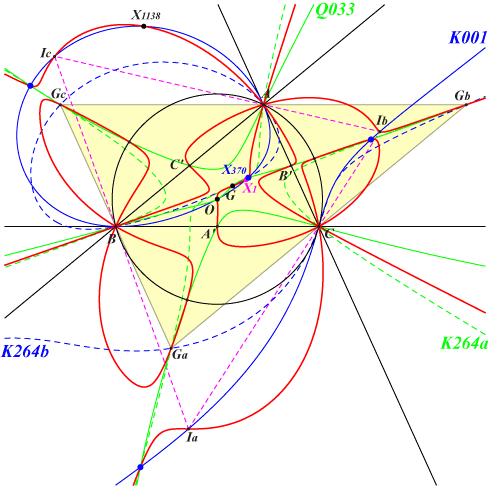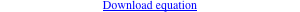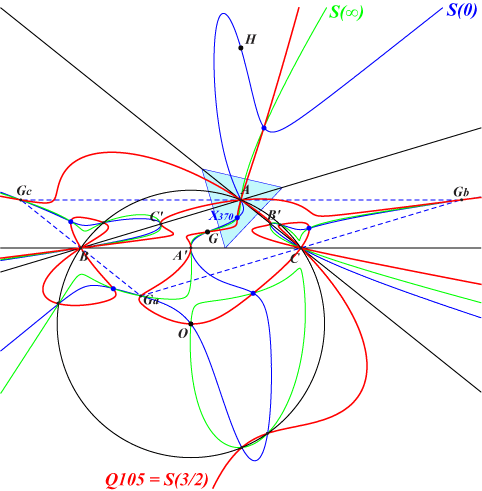too complicated to be written here. Click on the link to download a text file.X(1), X(2), X(3), X(370), X(1138) A'B'C' = medial triangle GaGbGc = antimedial triangle IaIbIc = excentral triangle five mates of X(370), see Table 10The locus of P such that the nine point center of the cevian triangle of P lies on the line OP is a septic passing through the vertices of the triangle ABC (which are triple points) and those of the medial, antimedial and excentral triangles (Angel Montesdeoca, private message, 2014-11-29 and also Anopolis #2003, Hatzipolakis). When the nine point center is replaced with the centroid (resp. the orthocenter) we obtain the quartic Q033 (resp. the quintic Q034). See a generalization below. Q105 meets the Neuberg cubic K001 at 21 points namely the vertices of ABC (counting for 9), the 4 in/excenters, the 6 X(370)-points, X(3), X(1138). Q105 meets Q033 at 28 points namely the vertices of ABC (counting for 9), the 6 X(370)-points, the 6 vertices of the medial and antimedial triangles, X(2), X(3) and 5 points on the circumcircle. Generalization Let G' and O' be the centroid and the circumcenter of the cevian triangle of P. Define X' such that O'X' = k O'G' (vectors). The locus of P such that X' lies on the line OP is a (possibly decomposed) septic S(k) passing through the points mentioned above namely the vertices of ABC (each counting for 9), the 6 X(370)-points, the vertices of the medial triangle (each counting for 2), the vertices of the antimedial triangle, X(2), X(3) and 5 points on the circumcircle. Examples : S(1) = Q033 and the sidelines of ABC, S(3) = Q034 and the circumcircle, S(3/2) = Q105, S(0) contains X(4), S(3/4) contains X(6).When the triangle ABC is "flat" enough, all the points mentioned are real, in particular the 6 equilateral cevian points (blue points).